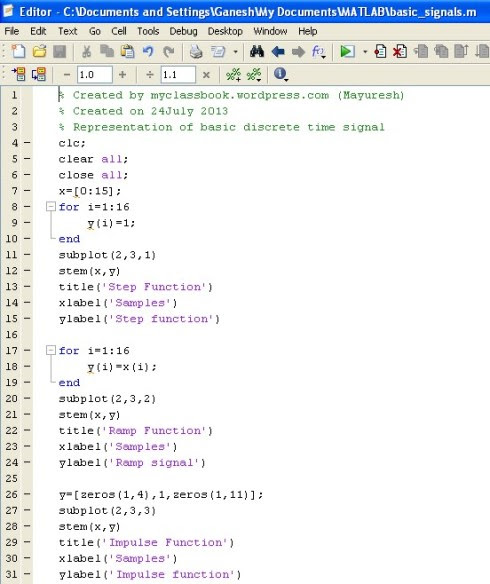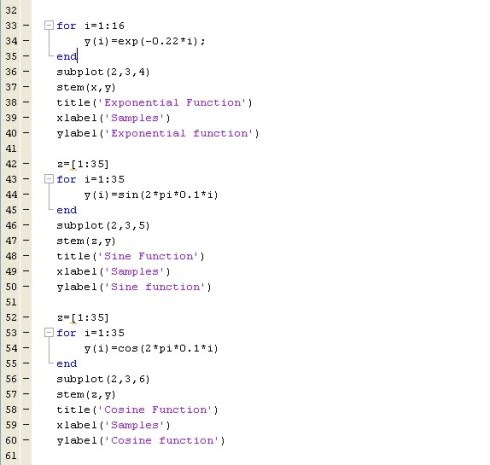# Representation of Basic Discrete Time Signal using MATLAB

Hi friends, today we are going to discuss discrete time signals and how to plot graphs of different discrete time signals such as step signal, a ramp signal, impulse function, exponential, sine and cosine signals using MATLAB.

Before going towards actual programming part, let us recall the definition of the discrete time signal.

“In discrete time signal, the value of the signal is specified only at a specific time. So signal represented at “discrete interval of time” is called as a discrete time signal.”

### MATLAB Program:

`clc;clear all;close all;x=[0:15];for i=1:16y(i)=1;endsubplot(2,3,1)stem(x,y)title('Discrete time Step Function')xlabel('Samples')ylabel('Step function')for i=1:16y(i)=x(i);endsubplot(2,3,2)stem(x,y)title('Discrete time Ramp Function')xlabel('Samples')ylabel('Ramp signal')y=[zeros(1,4),1,zeros(1,11)];subplot(2,3,3)stem(x,y)title('Discrete time Impulse Function')xlabel('Samples')ylabel('Impulse function')for i=1:16y(i)=exp(-0.22*i);endsubplot(2,3,4)stem(x,y)title('Discrete time Exponential Function')xlabel('Samples')ylabel('Exponential function')z=[1:35]for i=1:35y(i)=sin(2*pi*0.1*i)endsubplot(2,3,5)stem(z,y)title('Discrete time Sine Function')xlabel('Samples')ylabel('Sine function')z=[1:35]for i=1:35y(i)=cos(2*pi*0.1*i)endsubplot(2,3,6)stem(z,y)title('Discrete time Cosine Function')xlabel('Samples')ylabel('Cosine function')`

### Explanation:

• Representation of discrete time step function:

`x=[0:15];for i=1:16y(i)=1;endsubplot(2,3,1)stem(x,y)title('Discrete time Step Function')xlabel('Samples')ylabel('Step function')`

For representing discrete time step function, let us consider x-axis represents a number of samples and y-axis represents corresponding values of the signal. Here we use two matrix x and y. x=[0:15] represents matrix ‘x’ contains 16 elements from 0 to 15. Then we used one for loop from 1:16 (16 times) in which we define matrix y, which stores ‘1’ entire the matrix. Then we give command subplot(2,3,1). Here we used subplot command because we want all the basic signals on a single display. (2,3,1) represents there are 2 rows and 3 columns of graphs (it means 2*3=6 graphs are possible) 1 indicates that we want this graph at first position.

As we know for continuous signal plotting we use plot command but for a discrete time signal, we have to use stem command.

• Similarly, for discrete time ramp signal, we use y(i)=x(i) keeping all the program same.

• For impulse function we use y=[zeros(1,4),1,zeros(1,11)] that means first 4 elements of the matrix are ‘0(zeros)’ 5th element is ‘1’ and from 6th to 16th it is again ‘0’. Thus y becomes 16 element matrix. Thus we can plot a graph of impulse function using Matlab.

• For exponential signal, we have used exp(-0.22*i). Here negative sign indicates that the nature of the exponential signal is decreasing. But if you remove the negative sign it will become a simple increasing exponential signal.

• Similarly, we can plot discrete time sine and cosine functions using MATLAB

## MATLAB Program### You may also like

1.